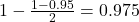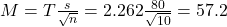## A shipment of 20,000 bags of oranges arrives at a distribution center with a nominal weight of 3200 g per bag. A sample of 10 bags is select

Question

A shipment of 20,000 bags of oranges arrives at a distribution center with a nominal weight of 3200 g per bag. A sample of 10 bags is selected and weighed. The average weight of the sample is 3300g, and the standard deviation of weight among cartons is 80 g. Find a 95% confidence interval for the mean weight of all the bags of oranges in the shipment.

in progress 0
2 months 2021-08-30T21:09:10+00:00 1 Answers 0 views 0

The 95% confidence interval for the mean weight of all the bags of oranges in the shipment is between 3242.8g and 3357.2g

Step-by-step explanation:

We have the standard deviation of the sample, so we use the t-distribution to solve this question.

T interval

The first step to solve this problem is finding how many degrees of freedom, we have. This is the sample size subtracted by 1. So

df = 10 – 1 = 9

95% confidence interval

Now, we have to find a value of T, which is found looking at the t table, with 9 degrees of freedom(y-axis) and a confidence level of. So we have T = 2.262

The margin of error is:In which s is the standard deviation of the sample and n is the size of the sample.

The lower end of the interval is the sample mean subtracted by M. So it is 3300 – 57.2 = 3242.8g

The upper end of the interval is the sample mean added to M. So it is 3300 + 57.2 = 3357.2g

The 95% confidence interval for the mean weight of all the bags of oranges in the shipment is between 3242.8g and 3357.2g